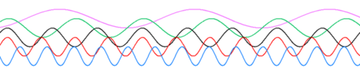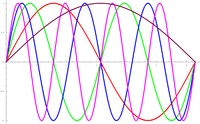## FANDOM

655 PagesSine waves of various frequencies; the lower waves have higher frequencies than those above.A better picture of Sine waves of different frequencies

Frequency is the measurement of the number of times that a repeated event occurs per unit of time. It is also defined as the rate of change of phase of a sinusoidal waveform. This in particular refers to Electric power supply in engineering.

## MeasurementEdit

To calculate the frequency of an event, the number of occurrences of the event within a fixed time interval are counted, and then divided by the length of the time interval.

In SI units, the result is measured in hertz (Hz), named after the German physicist Heinrich Rudolf Hertz. 1 Hz means that an event repeats once per second, 2 Hz is twice per second, and so on. This unit was originally called a cycle per second (cps), which is still used sometimes. Other units that are used to measure frequency include revolutions per minute (rpm) and radians per second (rad/s). Heart rate  and musical tempo  are measured in beats per minute (BPM).

An alternative method to calculate frequency is to measure the time between two consecutive occurrences of the event (the period) and then compute the frequency as the reciprocal of this time:

$f = \frac{1}{T}$

where T is the period. A more accurate measurement takes many cycles into account and averages the period between each.

## Frequency of wavesEdit

Measuring the frequency of sound, electromagnetic waves (such as radio or light), electrical signals, or other waves, the frequency in hertz is the number of cycles of the repetitive waveform per second. If the wave is a sound, frequency is what mainly characterizes its pitch.

Frequency has an inverse relationship to the concept of wavelength. The frequency f is equal to the speed v of the wave divided by the wavelength λ (lambda) of the wave:

$f = \frac{v}{\lambda}$

In the special case of electromagnetic waves moving through a vacuum, then v = c, where c is the speed of light in a vacuum, and this expression becomes:

$f = \frac{c}{\lambda}$

Note. When waves travel from one medium to another, their frequency remains exactly the same — only their wavelength and speed change.

## InvarianceEdit

Apart from its being modified by Doppler effect , frequency is an invariant quantity in the universe. That is, it cannot be changed by any physical process unlike velocity of propagation or wavelength.

## Reference frequenciesEdit

There are universally agreed reference frequencies for the measuring of time. One such method, used in atomic clocks, is based on the frequency of the Caesium  atom.

## ExamplesEdit

• The frequency of the standard pitch A above middle C  is usually defined as 440 Hz, that is, 440 cycles per second (Template:Audio) and known as concert pitch, to which an orchestra  tunes.
• A baby can hear tones with oscillations up to approximately 20,000 Hz, but these frequencies become more difficult to hear as people age.
• In Europe, the frequency of the alternating current in mains is 50 Hz (close to the tone G), however, in North America, the frequency of the alternating current is 60 Hz (close to the tone B flat — that is, a minor third  above the European frequency). The frequency of the 'hum' in an audio recording can show where the recording was made — in Europe or in America.

##Edit

Community content is available under CC-BY-SA unless otherwise noted.